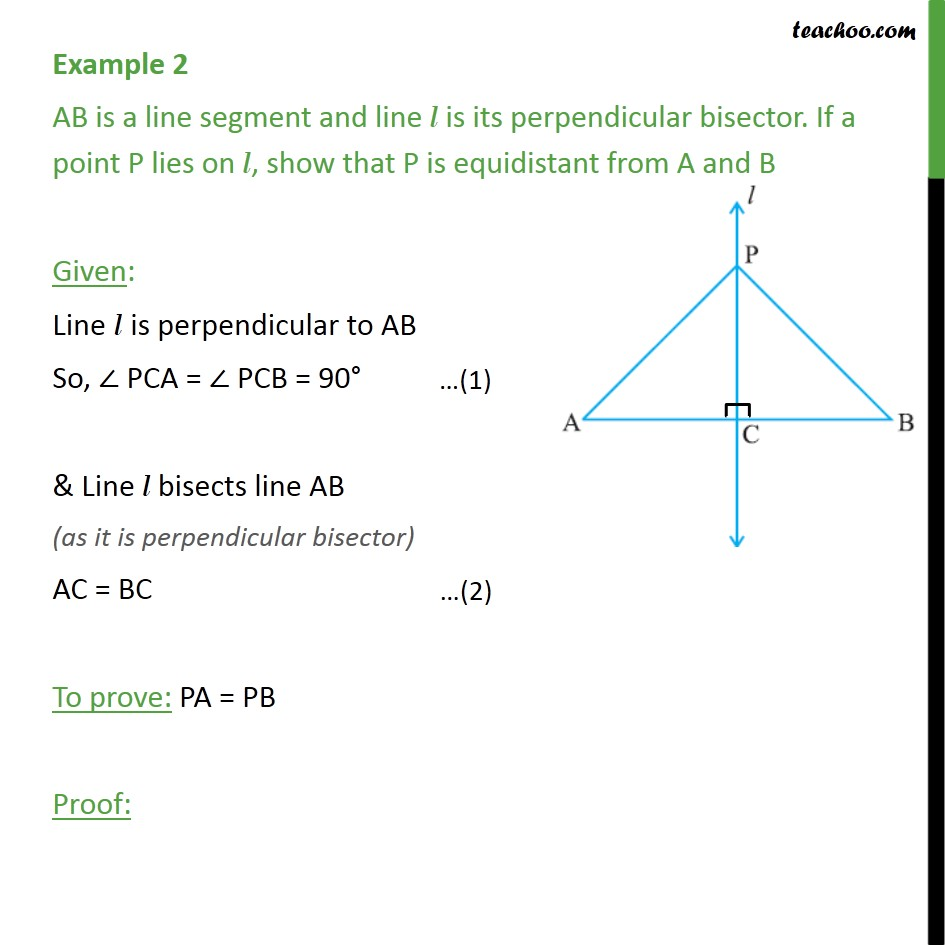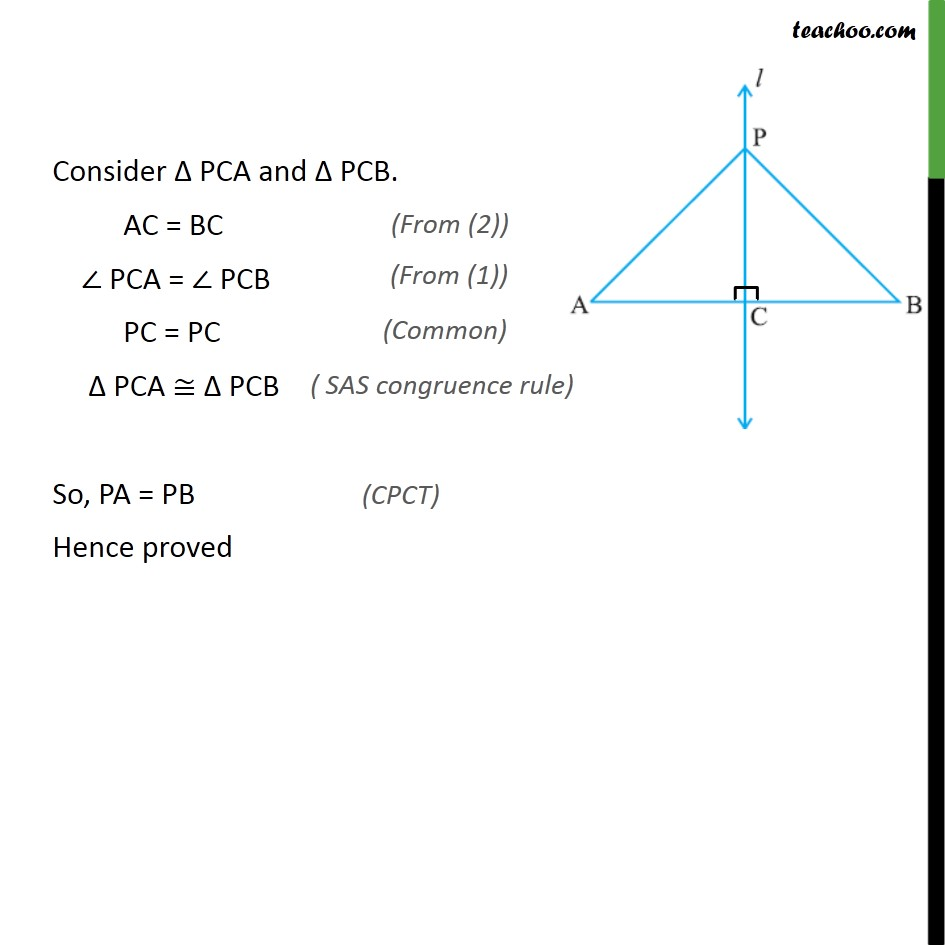Examples

Chapter 7 Class 9 Triangles
Serial order wiseLearn in your speed, with individual attention - Teachoo Maths 1-on-1 Class

### Transcript

Example 2 AB is a line segment and line l is its perpendicular bisector. If a point P lies on l, show that P is equidistant from A and B Given: Line l is perpendicular to AB So, PCA = PCB = 90 & Line l bisects line AB (as it is perpendicular bisector) AC = BC To prove: PA = PB Proof: Consider PCA and PCB. AC = BC PCA = PCB PC = PC PCA PCB So, PA = PB Hence proved